# Exercises: Chapter 5, Section 3

1. If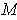is an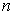-dimensional manifold (or manifold-with-boundary) in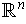, with the usual orientation, show that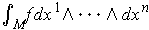, as defined in this section, is the same as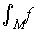, as defined in Chapter 3.

We can assume in the situation of Chapter 3 thathas the usual orientation. The singular-cubes with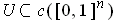can be taken to be linear maps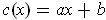where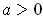and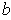are scalar constants. One has with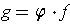, that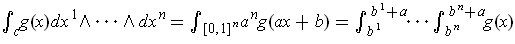. So, the two integrals give the same value.

1. Show that Theorem 5-5 is false ifis not required to be compact.

For example, if we letbe the open interval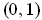, one has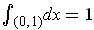but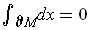. One can also let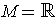and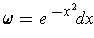.

2. Show that Theorem 5-5 holds for noncompactprovided that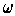vanishes outside of a compact subset of.

The compactness was used to guarantee that the sums in the proof were finite; it also works under this assumption because all but finitely many summands are zero ifvanishes outside of a compact subset of.

2. Ifis a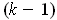-form on a compact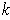-dimensional manifold, prove that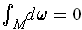. Give a counter-example ifis not compact.

One has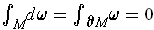as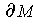is empty. Withthe set of positive real numbers, one has with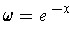that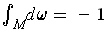.

3. An absolute-tensor on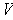is a function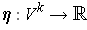of the form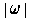for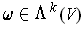. An absolute-form onis a function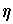such that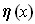is an absolute-tensor on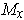. Show that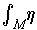can be defined, even ifis not orientable.

Make the definition the same as done in the section, except don't require the manifold be orientable, nor that the singular-cubes be orientation preserving. In order for this to work, we need to have the argument of Theorem 5-4 work, and there the crucial step was to replace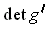with its absolute value so that Theorem 3-13 could be applied. In our case, this is automatic because Theorem 4-9 gives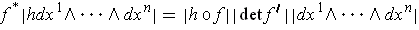.

4. If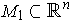is an-dimensional manifold-with-boundary and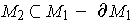is an-dimensional manifold with boundary, and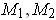are compact, prove that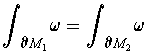whereis an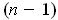-form on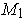, and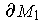and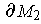have the orientations induced by the usual orieentations ofand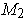.

Following the hint, let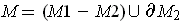. Thenis an-dimensional manifold-with-boundary and its boundary is the union ofand. Because the outward directed normals at points ofare in opposite directions forand, the orientation ofare opposite in the two cases. By Stokes' Theorem, we have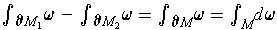. So the result is equivalent to. So, the result, as stated, is not correct; but, for example, it would be true ifwere closed.